# CBSE Solutions for Class 10 English

#### GSEB std 10 science solution for Gujarati check Subject Chapters Wise::

Identify whether they have One Solution, Infinite Solutions or No Solutions :-

3y+4x=6 , 12y+16x=30

જવાબ : Infinite Solutions

Identify whether they have One Solution, Infinite Solutions or No Solutions :- y+2x=10 , 3y+6x=30

જવાબ : One Solution

Identify whether they have One Solution, Infinite Solutions or No Solutions :- 7y−11x=53 ,19y−17x=456

જવાબ : No Solutions

Identify whether they have One Solution, Infinite Solutions or No Solutions :- y=7,x=−2

જવાબ : One Solution

Identify whether they have One Solution, Infinite Solutions or No Solutions :- ay+bx=a−b , by−ax=a+b

જવાબ : One Solution

Identify whether they have One Solution, Infinite Solutions or No Solutions :- 2y+3x=0, 124y+13x=0

જવાબ : One Solution

Identify whether they have One Solution, Infinite Solutions or No Solutions :- x=11 ,x=−11

જવાબ : One Solution

Identify whether they have One Solution, Infinite Solutions or No Solutions :-  152y−378x=−74 , −378y+152x=−604

જવાબ : No Solutions

Using substitution method solve the equation :- x−2y+300=0, 6x−y−70=0

જવાબ : x=2y−300 ----(1)
6x−y−70=0----(2)
Substituting value from (1) to (2)
6(2y−300)−y−70=06(2y−300)−y−70=0
=> y=170y=170
Putting this in (1)
x=40

Using substitution method solve the equation :- 5x−y=5, 3x−y=3

જવાબ : 5x−y=5 ----(1)
3x−y=3 ----(2)
Substituting value from (1) to (2)
3x + 5 -5x = 3
->   x=1
Putting this in (1)
->    y=0

Using elimination method solve the equation :- x+y−40= ,7x+3y=180

જવાબ : x+y−40=0  ---(A)
7x+3y=180  ---(B)
Multiplying equation (A) by 7
7x+7y−280=0 ---(C)
Subtracting equation (B) from equation (C)
We get
4y=100 => y=25
Substituting this in (A) ,we get x=15

Identify whether statement True or False :- 2x+3y=10 & 9x+11y=12 are consistent pair

જવાબ : True

Identify whether statement True or False :- 51x+68y=110 & 3x+4y=99 are consistent pair

જવાબ : False

Identify whether statement True or False :- x−3y=3 & 3x−9y=2 are consistent pair

જવાબ : False

Identify whether statement True or False :- x+6y=12 and 4x+24y=64 are inconsistent pair of equation

જવાબ : True

Identify whether statement True or False :-  2x+6y=12 and 8x+24y=65 are consistent pair of equation

જવાબ : False

Identify whether statement True or False :-  Lines represented by x−y=0 and x+y=0 are perpendicular to each other

જવાબ : True

Identify whether statement True or False :-   x=0 ,y=0 has one unique solution

જવાબ : True

Identify whether statement True or False :-  There are infinite solution for equation 17x+12y=30

જવાબ : True

Identify whether statement True or False :-  Pair of lines 117x+14y=30 , 65x+11y=19 are consistent and have a unique solution

જવાબ : True

Identify whether statement True or False :-  Line 4x+5y=0 and 11x+17y=0 both passes through origin

જવાબ : True

find the value of K for which the linear pair has infinite solution
12x+14y=0
36x+Ky=0

જવાબ : 64

There are 10 students in XII class. Some are maths and some bio student. The no of bio students are 4 more then math’s students. Find the no of math’s and bio students

જવાબ : Let x be math’s students
y be bio students
Then
x+y=10
y=x+4
Solving these linear pair through any method we get
x=3 and y=7

There are two numbers. Two conditions are there for them
(i) Sum of these two numbers are 100
(ii) One number is four time another number.
What are these numbers?

જવાબ : Let x and y are the number
x+y=100x+y=100
y=4xy=4x
Solving them we get x=20 and y=80

If 2x + 3y = 12 and 3x - 2y = 5 then solve the equation

જવાબ : x = 3, y = 2

If 4x+6y=3xy and 8x+9y=5xy then solve the equation

જવાબ : x=3, y=4

If 29x+37y=103 and 37x+29y=95 then solve the equation

જવાબ : x=2, y=1

If 2x + y = 2x – y = √8 then solve the equation

જવાબ : 0

The system kx - y = 2 and 6x - 2y = 3  has a unique solution only when k ≠ ____

જવાબ : 3

The system x - 2y = 3 and 3x + ky = 1 has a unique solution only when k ≠ ____

જવાબ : -6

The system x+2y=3 and 5x+ky+7=0 has no solution, when k = _____

જવાબ : 10

If the lines given by 3x + 2ky = 2 and 2x + 5y + 1 = 0 are parallel then the value of k is ____

જવાબ : 15/4

For what value of k do the equations kx - 2y = 3 and 3x + y = 5 represent two lines intersecting at a unique point?

જવાબ : k ≠ -6

The pair of equations x + 2y + 5 = 0 and -3x - 6y + 1 = 0 has _____ solution

જવાબ : no

The pair of equations 2x + 3y = 5 and 4x + 6y = 15 has _____ solution

જવાબ : no

Find the value of k for which the system of equations 3x+y=1 and kx+2y=5 has no solution.

જવાબ : k=6

Find the value of k for which the system of equations 3x+y=1 and kx+2y=5 has  a unique solution.

જવાબ : k≠-6

If x = -y and y > 0, The statement is correct and wrong :- 1/x – 1/y = 0

જવાબ : Wrong

If x = -y and y > 0, The statement is correct and wrong :- xy < 0

જવાબ : Correct

If x = -y and y > 0, The statement is correct and wrong :- x + y = 0

જવાબ : Correct

If x = -y and y > 0, The statement is correct and wrong :- x2y > 0

જવાબ : Correct

The graphic representation of the equations x+2y=3 and 2x+4y+7=0 gives a pair of ________  lines.

જવાબ : parallel

The graphs of the equations 2x+3y-2=0 and x-2y-8=0 are two lines which are

જવાબ : intersecting exactly at one point

The line of the equations 6x - 2y + 9 = 0 and 3x - y + 12 = 0 are ________

જવાબ : parallel

The sum of the digits of a two-digit number is 15. The number obtained by interchanging the digits exceeds the given number by 9. The number is _____

જવાબ : 40 years

The sum of the digits of a two-digit number is 15. The number obtained by interchanging the digits exceeds the given number by 9. The number is

જવાબ : 78

In a cyclic quadrilateral ABCD, it is being given that
∠A = (x + y + 10) °, ∠B = (y + 20) °,
∠C = (x + y - 30)° and ∠D = (x + y)°. Then, ∠B = ?

જવાબ : 80°

In a ΔABC, ∠C = 3 ∠B = 2 (∠A + ∠B), then ∠B = ?

જવાબ : 20°

If a pair of linear equations is inconsistent then their graph lines will be ______

જવાબ : parallel

If a pair of linear equations is consistent then their graph lines will be _____

જવાબ : intersecting or coincident

A two digit number is seven times the sum of its digits. The number formed by reversing the digits is 18 less than the given number. Find the given number. (2013)

જવાબ : Let unit’s place digit be x and ten’s place digit be y.
Then original number = x + 10y
and reversed number = 10x + y
According to the Question,
x + 10y = 7(x + y)
x + 10y = 7x + 7y
⇒ 10y – 7y = 7x – x
⇒ 3y = 6x ⇒ y = 2x …(A)
(x + 10y) – (10x + y) = 18
x + 10y – 10x – y = 18
⇒ 9y – 9x = 180
⇒ y – x = 2 …[Dividing by 9
⇒ 2x – x = 2 …[From (A)
∴ x = 2
Putting the value of ‘x’ in (A), we get y = 2(2) = 4
∴ Required number = x + 10y
= 2 + 10(4) = 42

The age of the father is twice the sum of the ages of his 2 children. After 20 years, his age will be equal to the sum of the ages of his children. Find the age of the father. (2012)

જવાબ : Let the present ages of his children be x years and y years.
Then the present age of the father = 2(x + y) …(A)
After 20 years, his children’s ages will be
(x + 20) and (y + 20) years
After 20 years, father’s age will be 2(x + y) + 20
According to the Question,
2(x + y) + 20 = x + 20 + y + 20
2x + 2y + 20 = x + y + 40
2x + 2y – x – y = 40 – 20
x + y = 20 …[From (A)
Present age of father = 2(20) = 40 years

Sita Devi wants to make a rectangular pond on the road side for the purpose of providing drinking water for street animals. The area of the pond will be decreased by 3 square feet if its length is decreased by 2 ft. and breadth is increased by 1 ft. Its area will be increased by 4 square feet if the length is increased by 1 ft. and breadth remains same. Find the dimensions of the pond. (2014)

જવાબ : Let, length of rectangular pond = x
breadth of rectangular pond = y
Area of rectangular pond = xy
According to Question,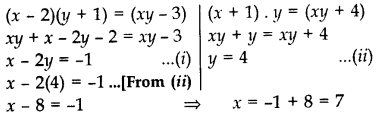=>  Length of rectangular pond = 7 ft.
Breadth of rectangular pond = 4 ft.

On reversing the digits of a two digit number, number obtained is 9 less than three times the original number. If difference of these two numbers is 45, find the original number. (2014)

જવાબ : Let unit’s place digit be x and ten’s place digit bey.
Original number = x + 10y Reversed number = 10x + y
According to the Question,
10x + y = 3(x + 10y) – 9

10x + y = 3x + 30y – 9
10x + y – 3x – 30y = -9
7x – 29y = -9 …(A)
10x + y – (x + 10y) = 45

9x – 9y = 45
x – y = 5 …[Dividing both sides by 9
x – 5 + y …(B)
Solving (
A),
7x – 29y = -9
7(5 + y) – 29y = -9 …[From (B)
35+ 7y – 29y = -9
-22y = -9 – 35
-22y = -44
y = 44/22 = 2
Putting the value of y in (B),
x = 5 + 2 = 7

Original number = x + 10y
= 7 + 10(2) = 27

Speed of a boat in still water is 15 km/h. It goes 30 km upstream and returns back at the same point in 4 hours 30 minutes. Find the speed of the stream. 2017D

જવાબ : Let, speed of stream = x km/hr
Speed of boat in still water = 15 km/hr
then, the speed of the boat upstream = (15 – x) km/hr
and the speed of the boat downstream = (15 + x) km/hr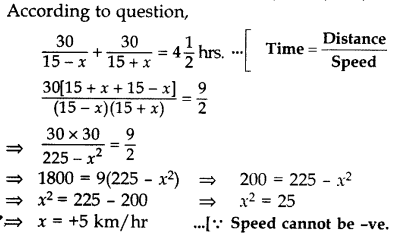=> Speed of stream = 5 km/hr

The owner of a taxi company decides to run all the taxis on CNG fuel instead of petrol/diesel. The taxi charges in city comprises of fixed charges together with the charge for the distance covered. For a journey of 12 km, the charge paid is 789 and for journey of 20 km, the charge paid is ₹145.
What will a person have to pay for travelling a distance of 30 km? (2014)

જવાબ : Let the fixed charges = 7x
and the charge per km = ₹y
According to the Question,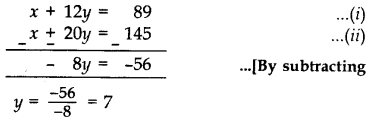Putting the value of y in (A), we get
x + 12(7) = 89
x + 84 = 89 x = 89 – 84 = 5
Total fare for 30 km = x + 30y = 5 + 30(7)
= 5 + 210 = ₹215

A boat takes 4 hours to go 44 km downstream and it can go 20 km upstream in the same time. Find the speed of the stream and that of the boat in still water. (2015)

જવાબ : Let the speed of the stream = y km/hr
Let the speed of boat in still water = x km/hr
then, the speed of the boat in downstream = (x + y) km/hr
and, the speed of the boat in upstream = (x – y) km/hr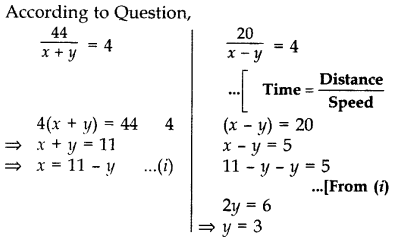From (A), x = 11 – 3 = 8
Speed of the stream, y =3 km/hr
Speed of the boat in still water, x = 8 km/hr

A man travels 300 km partly by train and partly by car. He takes 4 hours if the travels 60 km by train and the rest by car. If he travels 100 km by train and the remaining by car, he takes 10 minutes longer. Find the speeds of the train and the car separately. (2017D)

જવાબ : Let, the speed of train = x km/hr
the speed of car = y km/ hr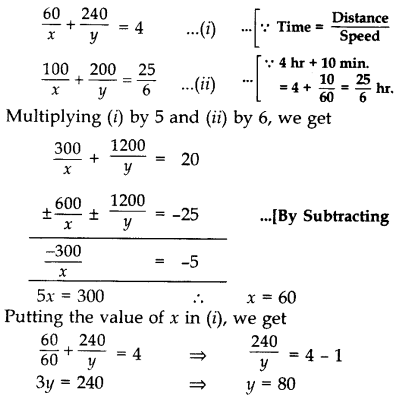=>  Speed of the train = 60 km/hr
and Speed of the car = 80 kn/hr

The owner of a taxi company decides to run all the taxis on CNG fuel instead of petrol/diesel. The taxi charges in city comprises of fixed charges together with the charge for the distance covered. For a journey of 13 km, the charge paid is ₹129 and for a journey of 22 km, the charge paid is ₹210.
What will a person have to pay for travelling a distance of 32 km? (2014 )

જવાબ : Let fixed charge be ₹x and the charge for the distance = ₹y per km
According to the Question,
For a journey of 13 km,
x + 13y = 129 x = 129 – 13y …(A)
For a journey of 22 km, x + 22y = 210 …(B)
129 – 13y + 22y = 210 …[From (A)
9y = 210 – 129 = 81
9y = 81 y = 9
From (A), x = 129 – 13(9)
= 129 – 117 = 12
Fixed charge, x = ₹12
The charge for the distance, y = ₹9 per km
To pay for travelling a distance of 32 km
= x + 32y = 12 + 32(9) = 12 + 288 = ₹300

Solve the following pair of linear equations graphically:
x + 3y = 6 ; 2x – 3y = 12
Also find the area of the triangle formed by the lines representing the given equations with y-axis. (2012, 2015)

જવાબ :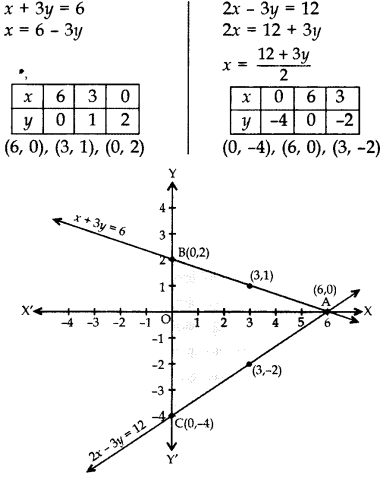By plotting points and joining them, the lines intersesct at A(6, 0)
x = 6, y = 0
Line x + 3y = 6 intersects Y-axis at B(0, 2) and Line 2x – 3y = 12 intersects Y-axis at C(0, -4). Therefore, Area of triangle formed by the lines with y-axis.
Area of triangle
= ½ base × corresponding altitude
= ½ × BC × AO = ½ × 6 × 6 = 18 sq. units

Draw the graphs of following equations:
2x – y = 1; x + 2y = 13
Find the solution of the equations from the graph and shade the triangular region formed by the lines and the y-axis. (2013)

જવાબ :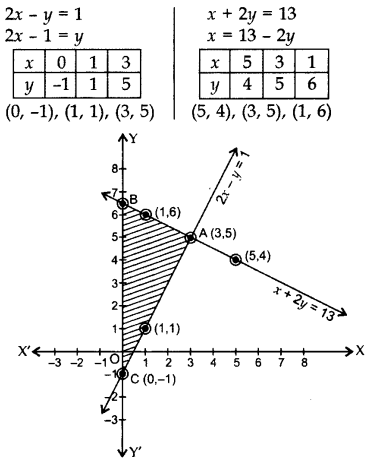By plotting the points and joining the lines, they intersect at A(3,5).
=>  x = 3, y = 5
∆ABC is the required triangle.

Draw the graphs of the equations x – y + 1 = 0 and 3x + 2y – 12 = 0. Determine the coordinates of the vertices of the triangle formed by these lines and x-axis. (2012, 2017D)

જવાબ :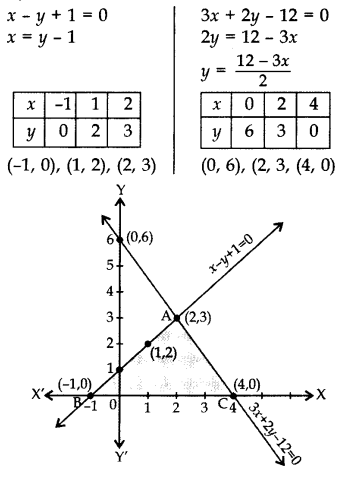Lines intersect at (2, 3)

=> x = 2, y = 3
A(2, 3), B(-1, 0) and C(4, 0) are Vertices of ∆ABC

Amit bought two pencils and three chocolates for ₹11 and Sumeet bought one pencil and two chocolates for ₹7. Represent this situation in the form of a pair of linear equations. Find the price of one pencil and that of one chocolate graphically. (2017OD)

જવાબ : Let the price of one pencil = ₹x and the price of one chocolate = ₹y.
As per the Question,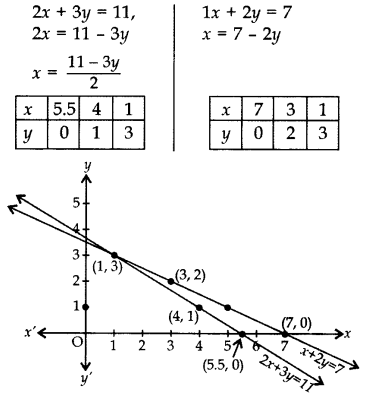Lines intersect at (1, 3).
=>  x = 1, y = 3
Therefore the price of one pencil = ₹1

& price of one chocolate = ₹3

7x – 5y – 4 = 0 is given. Write another linear equation, so that the lines represented by the pair are:
(i) intersecting
(ii) coincident
(iii) parallel (2015 OD)

જવાબ : 7x – 5y – 4 = 0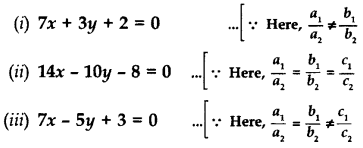The cost of 2 kg of orange and 1kg of grapes on a day was found to be Rs.160. After a month, the cost of 4 kg of oranges and 2 kg of grapes is Rs.300. Represent the situation algebraically.

જવાબ : Let the cost of 1 kg of oranges be ‘Rs. x’.
And, let the cost of 1 kg of grapes be ‘Rs. y’.
According to the question, the algebraic representation is
2x + y = 160
And 4x + 2y = 300

For, 2x + y = 160 or y = 160 − 2x, the solution table is;

 X 50 60 70 Y 60 40 20
For 4x + 2y = 300 or y = (300 – 4x)/ 2, the solution table is;

 X 70 80 75 Y 10 -10 0

Half the perimeter of a rectangular garden, whose length is 4 m more than its width, is 36 m. Find the dimensions for f the garden.

જવાબ : Given, half the perimeter of a rectangular garden = 36 m

so, 2(l + b)/2 = 36

(l + b) = 36 ……….(1)
Given, the length is 4 m more than its width.

Let width = x

And length = x + 4

Substituting this in eq(1), we get;

x + x + 4 = 36
2x + 4 = 36
2x = 32
x = 16

Therefore, the width is 16 m and the length is 16 + 4 = 20 m.

On comparing the ratios a1/a2, b1/b2, and c1/c2, find out whether the following pair of linear equations are consistent, or inconsistent.

(i) 3x + 2y = 5 ; 2x – 3y = 7

(ii) 2x – 3y = 8 ; 4x – 6y = 9

જવાબ : (i) Given : 3x + 2y = 5 or 3x + 2y – 5 = 0
and 2x – 3y = 7 or 2x – 3y – 7 = 0

Comparing the above equations with a1x + b1y + c1=0
And a2x + b2y + c2 = 0
We get,
a= 3, b= 2, c= -5
a= 2, b= -3, c= -7

a1/a= 3/2, b1/b= 2/-3, c1/c= -5/-7 = 5/7
Since, a1/a2≠b1/bthe lines intersect each other at a point and have only one possible solution.

Hence, the equations are consistent.

(ii) Given 2x – 3y = 8 and 4x – 6y = 9
Therefore,
a= 2, b= -3, c= -8
a= 4, b= -6, c= -9

a1/a= 2/4 = 1/2, b1/b= -3/-6 = 1/2, c1/c= -8/-9 = 8/9

Since, a1/a2=b1/b2≠c1/c2

Therefore, the lines are parallel to each other and they have no possible solution. Hence, the equations are inconsistent.

Solve the following pair of linear equations by the substitution method.

(i) x + y = 14
x – y = 4

(ii) 3x – y = 3
9x – 3y = 9

જવાબ : (i) Given,
x + y = 14 and x – y = 4 are the two equations.
From 1st equation, we get,
x = 14 – y
Now, put the value of x in second equation to get,
(14 – y) – y = 4
14 – 2y = 4
2y = 10
Or y = 5
By the value of y, we can now find the value of x;
x = 14 – y
x = 14 – 5
Or x = 9
Hence, x = 9 and y = 5.

(ii) Given,
3x – y = 3 and 9x – 3y = 9 are the two equations.
From 1st equation, we get,
x = (3 + y)/3
Now, substitute the value of x in the given second equation to get,
9[(3 + y)/3] – 3y = 9

3(3+y) – 3y = 9
9 + 3y – 3y = 9
9 = 9
Therefore, y has infinite values and since, x = (3 + y)/3, so x also has infinite values.

Solve 2x + 3y = 11 and 2x – 4y = – 24 and hence find the value of ‘l’ for which y = lx + 3.

જવાબ : 2x + 3y = 11…………………………..(i)
2x – 4y = -24………………………… (ii)
From equation (ii), we get;
x = (11 – 3y)/2 ……….…………………………..(iii)

Putting the value of x in equation (ii), we get

2[(11 – 3y)/2] – 4y = −24
11 – 3y – 4y = -24
-7y = -35
y = 5……………………………………..(iv)
Putting the value of y in equation (iii), we get;

x = (11 – 15)/2 = -4/2 = −2
Hence, x = -2, y = 5
Also,
y = lx + 3
5 = -2l +3
-2l = 2
l = -1
Therefore, the value of l is -1

The coach of a cricket team buys 7 bats and 6 pair of pads for Rs.3800. Later, she buys 3 bats and 5pair of pads for Rs.1750. Find the cost of each bat and each pair of pad.

જવાબ : Let the cost of a bat be x and the cost of a pair of pad be y.
According to the question,
7x + 6y = 3800 ………………. (i)
3x + 5y = 1750 ………………. (ii)
From (i), we get;
y = (3800 – 7x)/6 …………………… (iii)
Substituting (iii) in (ii). we get,
3x + 5[(3800 – 7x)/6] = 1750
3x + (9500/3) – (35x/6) = 1750
3x – (35x/6) = 1750 – (9500/3)

(18x – 35x)/6 = (5250 – 9500)/3
-17x/6 = -4250/3
-17x = -8500
x = 500
Putting the value of x in (iii), we get;

y = (3800 – 7 × 500)/6 = 300/6 = 50
Hence, the cost of a bat is Rs 500 and cost of pair of pad is Rs 50

### There are No Content Availble For this Chapter

Coordinates of points on line x+y=0

 Co-ordintes of x Co-ordinates of y 1 1 A -7 2 3 B -1 3 5 C -3 4 7 D -5

જવાબ :

1-B, 2-C, 3-D, 4-A

Coordinates of points on line x-y=0

 Co-ordintes of x Co-ordinates of y 1 1 A 7 2 3 B 5 3 5 C 3 4 7 D 1

જવાબ :

1-D, 2-C, 3-B, 4-A

Coordinates of points on line 2x+y=0

 Co-ordintes of x Co-ordinates of y 1 4 A -4 2 -6 B -2 3 8 C 5 4 -10 D 3

જવાબ :

1-B,2-D,3-A, 4-C

Coordinates of points on line x+2y=0

 Co-ordintes of x Co-ordinates of y 1 -8 A 1 2 -2 B -2 3 6 C -3 4 4 D 4

જવાબ :

1-D, 2-A, 3-C, 4-B

Coordinates of points on line 2x-y=0

 Co-ordintes of x Co-ordinates of y 1 2 A 6 2 3 B 10 3 4 C 4 4 5 D 8

જવાબ :

1-C, 2-A, 3-D, 4-B

Coordinates of points on line 2x= -3y

 Co-ordintes of x Co-ordinates of y 1 -9 A 2 2 -3 B 4 3 -12 C 6 4 -6 D 8

જવાબ :

1-C, 2-A, 3-D, 4-B

Coordinates of points on line x-3y=0

 Co-ordintes of x Co-ordinates of y 1 6 A 1 2 9 B 2 3 12 C 3 4 3 D 4

જવાબ :

1-B, 2-C, 3-D, 4-A

Coordinates of points on line 2x-3y=0

 Co-ordintes of x Co-ordinates of y 1 3 A 8 2 6 B 6 3 9 C 4 4 12 D 2

જવાબ :

1-D, 2-C, 3-B, 4-A

Coordinates of points on line 2x-3y=0

 Co-ordintes of x Co-ordinates of y 1 3 A 8 2 6 B 6 3 9 C 4 4 12 D 2

જવાબ :

1-D, 2-C, 3-B, 4-A

Coordinates of points on line x-5y=0

 Co-ordintes of x Co-ordinates of y 1 40 A 5 2 25 B 6 3 35 C 7 4 30 D 8

જવાબ :

1-D, 2-A, 3-C, 4-B

### Take a Test

Choose your Test :

### Pair of Linear Equations in Two Variables

Math
Chapter 03 : Pair of Linear Equations in Two Variables

### Browse & Download CBSE Books For Class 10 All Subjects

The GSEB Books for class 10 are designed as per the syllabus followed Gujarat Secondary and Higher Secondary Education Board provides key detailed, and a through solutions to all the questions relating to the GSEB textbooks.

The purpose is to provide help to the students with their homework, preparing for the examinations and personal learning. These books are very helpful for the preparation of examination.

For more details about the GSEB books for Class 10, you can access the PDF which is as in the above given links for the same.